# Milliman Lecture Archives

## 2018-19 Milliman Lectures

###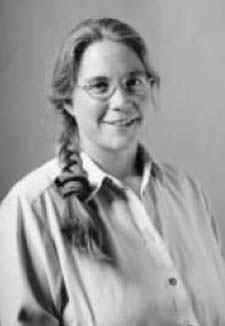Karen E. Smith

M. S. Keeler Professor of Mathematics
University of Michigan

A series of three lectures entitled Singularities in Algebraic Geometry: Resolving and Measuring using reduction modulo p

Lecture I - Resolutions of Singularities
While conic sections and spheres are smooth varieties, in general, a variety can have singular points—places where it is pinched or intersects itself. In this first talk, we will discuss Hironaka's famous theorem on resolution of singularities—a technique to "get rid" of the singular points. We introduce a class of singular varieties called rational singularities that are important because they are well-approximated by their resolutions, and explain how one can use "reduction modulo p" to characterize them.

Lecture II - Measuring Singularities
We discuss some ways to quantify how singular a given variety is. We introduce an analytic index of singularities, defined in terms of convergence of a certain integral. This provides a numerical measure of the singularity, sometimes called the log-canonical threshold. Remarkably, this numerical invariant can also be defined using reduction to characteristic p: for each p, we define a numerical measure of singularities called the F-pure threshold, which has striking fractal-like behavior. Amazing, taking the limit as p goes to infinity, we recover the analytically defined log canonical threshold. We will discuss these theorems and some deep open questions that remain at the frontier of this topic.

Lecture III - Non-Commutative Resolutions of Singularities
A foundational result of Hilbert underlies all of algebraic geometry: an algebraic variety can be understood more or less completely by fully understanding its ring of regular (polynomial) functions. As we saw in the first two lectures, questions about varieties can be translated into corresponding questions about their coordinate rings—which are always commutative Noetherian rings—and then attacked with tools of commutative algebra. On the other hand, many of these algebraic tools make sense even for non-commutative rings. In the final talk, we consider what it might mean to have a "non-commutative" variety, and what it might mean that it is "smooth." We will introduce Van den Bergh's idea of a non-commutative resolution of singularities, and again, show how working over a field of prime characteristic can provide insight into this problem.

Top of page

## 2017-18 Milliman Lectures

###Martin Hairer

Chair in Probability and Stochastic Analysis
Imperial College London

Lecture I - Bridging scales: from microscopic dynamics to macroscopic laws
One fascinating aspect of probability theory is the universal aspect of the objects it allows us to construct.  The most well-known example of this phenomenon is the central limit theorem: for a very large class of collections of random variables, additive functionals that only depend weakly on any one element of the collection exhibit Gaussian behaviour in the limit.  When taking time evolution into account, it turns out that in certain "cross-over regimes" the large-scale behaviour of a number of stochastic systems can formally be described by an ill-posed stochastic PDE.

Lecture II - An analyst’s incursion into quantum field theory
In quantum field theory, scattering amplitudes are described by sums over terms described by Feynman diagrams.  In many cases, these are meant to describe singular divergent integrals, so it is not clear how they should be interpreted and whether different interpretations are equivalent.  In the Euclidean context, we turn this into a clean problem of real analysis and show how algebraic techniques allow to tackle it.

Lecture III - Renormalization: a BPHZ theorem for stochastic PDEs
We show how some of the ideas explored in the previous two lectures can be applied to the study of singular stochastic PDEs.  In particular, we show that although it is not clear a priori what it means to "solve" any particular one of these equations, they can be divided into finite-dimensional families of solutions that are themselves defined in a canonical way.  This is a consequence of a version of the celebrated "BPHZ theorem" which governs renormalisation techniques in perturbative QFT.

Top of page

## 2016-2017 Milliman Lectures

###Fan Chung

Distinguished Professor of Mathematics and Computer Science
University of California, San Diego

Lecture I: Can you hear the shape of a network?
New directions in spectral graph theory We will discuss some recent developments in several new directions of spectral graph theory, including random walks for directed graphs, ranking algorithms, network games, graph limits and graphlets, for example.

Lecture II: 'Sequences: random, structured or something in between?'
There are many fundamental problems concerning sequences that arise in many areas of mathematics and computation. Typical problems include finding or avoiding patterns; testing or validating various 'random-like' behavior; analyzing or comparing different statistics, etc. In this talk, we will examine various notions of regularity or irregularity for sequences and mention numerous open problems.

Lecture III: Semigroup Spectral Theory and Graph Coloring Games
We consider a coloring game on graphs as an example to illustrate the effectiveness of the semigroup spectral method for determining the spectrum of the directed graphs which arise as the state graphs associated with the game. Originating from the study of the so-called Tsetlin library random walks and the like, this method can be used to analyze dynamic processes such as voting and ranking, provided the random processes satisfy certain "memoryless" conditions (corresponding to left-regular-band semigroups).

Top of page

## 2015-2016 Milliman Lectures

###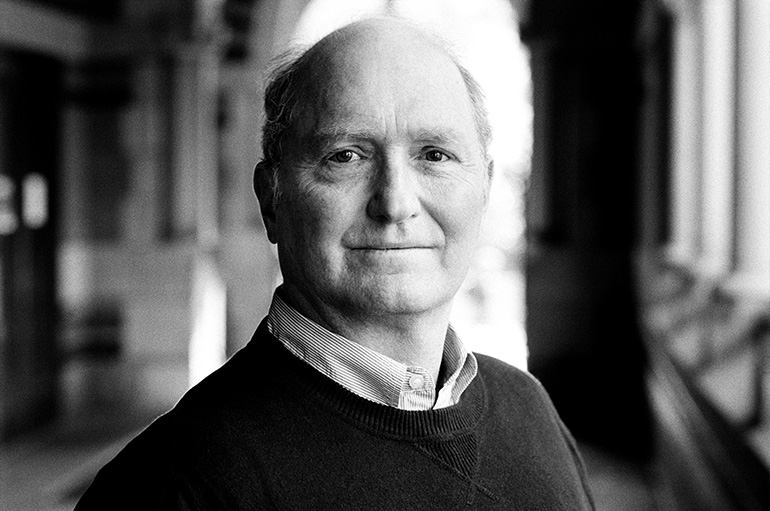Curtis McMullen

Cabot Professor of Mathematics
Harvard University

A series of three lectures presenting unexpected interactions between low-dimensional dynamics, complex analysis and algebraic geometry.

Lecture I: Billiards and Moduli Spaces
Video: https://youtu.be/Unb_TeVFwl8

Lecture II: Entropy, Integers and Algebraic Dynamics
Video: https://youtu.be/DXH-64KCnuo

Lecture III: Surfaces in the Space of Surfaces
Video: https://youtu.be/mSV5ytQMstE

Top of page

## 2015-2016 Milliman Lectures

###Laure Saint-Raymond

Professor of Mathematics
Pierre & Marie Curie University (Paris VI), and École Normale Supérieure

A series of three lectures entitled From Particle Systems to Kinetic Equations

Lecture I: The low density limit: formal derivation
Consider a deterministic system of N hard spheres of diameter ε. Assume that they are initially independent and identically distributed. Then, in the limit when N→∞ and ε→0 with Nεd-1=1 (Boltzmann-Grad scaling), the one-particle density can be approximated by the solution to the kinetic Boltzmann equation. In particular, particles remain asymptotically independent. In the first lecture, we will present the formal derivation of this low density limit, and discuss two important features, namely the propagation of chaos and the appearance of irreversibility.

Video: https://youtu.be/_8J-bWWZPAI

Lecture II: A short time convergence result
Lanford's theorem states that in the Boltzmann-Grad limit the one-particle density converges to the solution of the kinetic Boltzmann equation almost everywhere on a short time interval (corresponding actually to a fraction of the average first collision time). The proof relies on a careful study of the recollision mechanism (which is not described by the Boltzmann dynamics), and on a priori bounds obtained by a Cauchy-Kowalewski argument. In the second lecture, we will give a sketch of this proof, and show that the time restriction is due to the lack of global a priori bounds.

Video: https://youtu.be/S6JKI370gh0

Lecture III: The case of fluctuations around a global equilibrium
To improve the convergence time, the idea is therefore to consider situations where one can obtain global a priori bounds. Since the system of particles is Hamiltonian, the Gibbs measures are invariant under the flow and provide constant solutions to the Boltzmann equation. By perturbation, we get initial data leading to linear kinetic equations. In the third lecture, we will show that, for such initial data, we can control a priori the collision rate, and extend Lanford's strategy to get a quasi-global convergence result in the low density limit.

Video: https://youtu.be/_H3DxJBqWcw

Top of page

## 2014-2015 Milliman Lectures

###Richard M. Schoen

Distinguished Professor and Excellence in Teaching Endowed Chair
University of California, Irvine

Lecture I: Problems and progress in mathematical relativity
The Einstein equations were invented to unify gravity and special relativity. The theory of curved space times evolving under the Einstein evolution equations leads to a unique set of mathematical problems which lie in the fields of differential geometry and partial differential equations of elliptic, hyperbolic, and parabolic type. This lecture will survey some of these problems with emphasis on topics of current interest for which progress is being made. It is intended for a general audience.

Video: https://youtu.be/jBjOVFGEhZE

Lecture II: Two eigenvalue problems for surfaces
The problem of finding surface geometries (metrics) of a given area which maximize their lowest eigenvalue has been studied for over 50 years. Despite some spectacular successes the problem is still not well understood for most surfaces. In this colloquium lecture we will describe this question and the results which have been obtained. We will also describe a related problem for surfaces with boundary. Both of these questions lead to geometric descriptions of extremal metrics as minimal surfaces and thus lead to a new set of minimal surfaces questions.

Video: https://youtu.be/Tvs0Q_wrl4Y

Lecture III: Localizing solutions of the Einstein equations
In general it is not possible to localize solutions of the Einstein equations since there are asymptotic conserved quantities such as the total mass which are nonzero for every nontrivial space-time. In this lecture we will describe recent work with A. Carlotto which achieves a localization in cones and leads to new constructions of non-interacting superpositions of solutions.

Video: https://youtu.be/H7z6m1TzOt4

Top of page

## 2013-2014 Milliman Lectures

###Michael J. Hopkins

Professor of Mathematics
Harvard University

Lecture I: Chern-Weil theory and abstract homotopy theory
I will describe how abstract homotopy theory can be used to define and describe the natural invariants associated to a connection. The formulation recovers the classical Chern-Weil theory associating a characteristic class to an invariant polynomial on the Lie algebra.

Video: https://youtu.be/SAmHQu20gz4

Lecture II & III: Topological Path integrals (Ambidexterity? Analogies with K-theory?)
Many topological quantum field theories are constructed from a classical action which is topologically invariant in the sense that it does not involve metric terms. But so far there are no purely topological means of forming the path integral and thereby constructing the theory. In this talk I will discuss some tools coming from chromatic homotopy theory for approaching this problem.

Video: https://youtu.be/9DLwFhcEmYw
Video: https://youtu.be/RdYNabzzhLo

Top of page

## 2012-2013 Milliman Lectures

###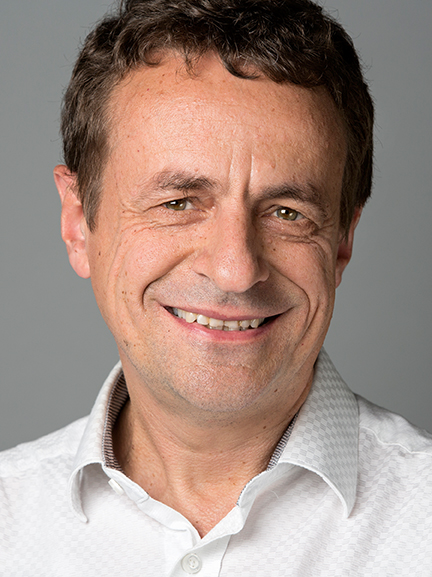Bernd Sturmfels

Professor of Mathematics, Statistics, and Computer Science
University of California, Berkeley

Lecture I: Convex Algebraic Geometry
We introduce convex bodies with an interesting algebraic structure. A primary focus lies on the geometry of semidefinite optimization. Starting with elementary questions about ellipses in the plane, we move on to discuss the geometry of spectrahedra, orbitopes, and convex hulls of real varieties. This lecture has many beautiful pictures and can be enjoyed by undergraduate students.

Video: https://youtu.be/MKv8-z4zyJ8

Lecture II: Maximum Likelihood for Matrices with Rank Constraint
Maximum likelihood estimation is a fundamental computational task in statistics. We discuss this problem for manifolds of low rank matrices. These represent mixtures of independent distributions of two discrete random variables. This non-convex optimization problems leads to some beautiful geometry, topology, and combinatorics. We explain how numerical algebraic geometry is used to find the global maximum of the likelihood function, and we present a remarkable duality theorem due to Draisma and Rodriguez.

Video: https://youtu.be/CM3hdOxpj10

Lecture III: Tropicalization of Classical Moduli Spaces
Algebraic geometry is the study of solutions sets to polynomial equations. Solutions that depend on an infinitesimal parameter are studied combinatorially by tropical geometry. Tropicalization works especially well for varieties that are parametrized by monomials in linear forms. Many classical moduli spaces (for curves of low genus and few points in the plane) admit such a representation, and we here explore their tropical geometry. Examples to be discussed include the Segre cubic, the Igusa quartic, the Burkhardt quartic, and moduli of marked del Pezzo surfaces. Matroids, hyperplane arrangements, and Weyl groups play a prominent role. Our favorites are E6, E7 and G32.

Video: https://youtu.be/WKoi5Mu2dsE

Top of page

## 2011-2012 Milliman Lectures

###Wendelin Werner

Professor of Mathematics
Université Paris-Sud

A series of three lectures entitled Aspects of Conformal Invariant Randomness

Lecture I: The Global Picture
In this lecture, I will try to give an overview of some of the main ideas and results in the general area of two-dimensional random models - including the discussion of conformal invariance of discrete models, Schramm's SLE processes and their generalizations, planar maps, and some open questions.

Video: https://youtu.be/acwabBW1xv4

Lecture II: Introduction to the Gaussian Free Field
In this lecture, I will give an introduction to the Gaussian Free Field, its properties and how it can be related to SLE processes, and Conformal Loop Ensembles.

Video: https://youtu.be/U7x65PhWyGY

Lecture III: Conformal Loop Ensembles
In this third lecture, I will focus more on some properties of the Conformal Loop Ensembles, including their precise relation to SLE and Brownian Loops.

Video: https://youtu.be/MB-tSD1gHEs

Top of page

## 2010-2011 Milliman Lectures

###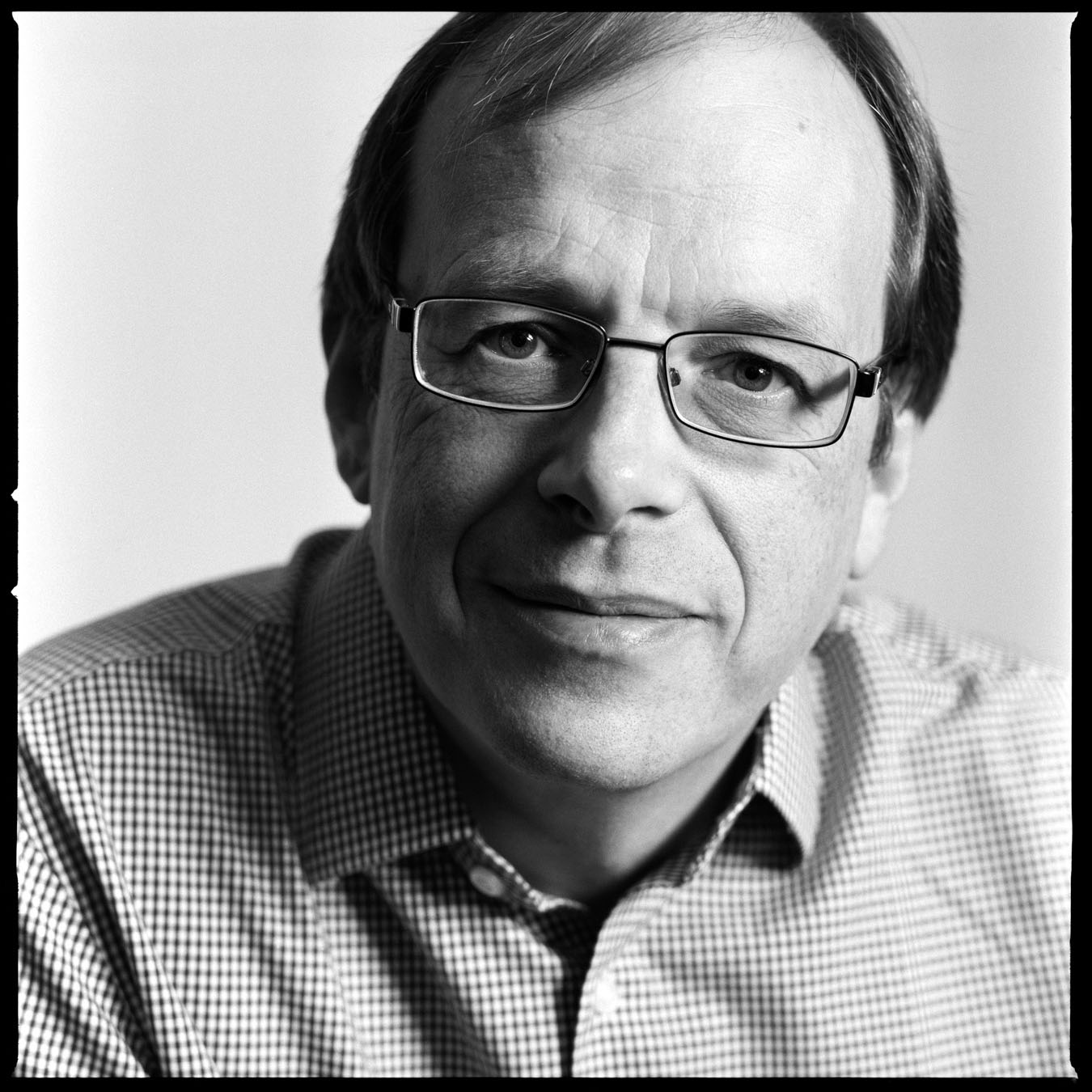Richard Taylor

Herchel Smith Professor of Mathematics
Harvard University

Lecture I: Reciprocity Laws and Density Theorems
If one fixes a polynomial (or a system of polynomials) in one or more variables, one can ask how the number of solutions modulo a prime number p varies with the prime p. Reciprocity laws give a formula involving completely different areas of mathematics (discrete subgroups of Lie groups). Density theorems give statistical information on how the number of solutions varies with p.
In the first lecture I will present a leisurely historical introduction to reciprocity laws and density theorems, starting with Gauss' law of quadratic reciprocity and finishing with the Sato-Tate conjecture. This lecture will also sketch Serre's approach to deducing density theorems from reciprocity laws.

Lecture II: Galois Representations
In the second lecture I will talk about a more general framework for discussing reciprocity laws. I will introduce Galois representations, L-functions and automorphic forms, and discuss their relevance in number theory. I will describe Langlands' very general reciprocity conjecture and the Fontaine-Mazur conjecture.

Lecture III: Automorphy
I will describe what we currently know about general reciprocity theorems. I will give some indication of the techniques we have and what I see as the main stumbling blocks to further progress.

Top of page

## 2009-2010 Milliman Lectures

###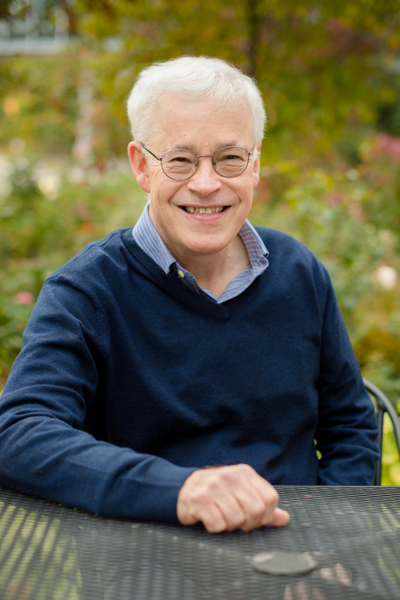Nick Trefethen

Professor of Numerical Analysis
Oxford University

Lecture I: Four Bugs on a Rectangle (and the Biggest Numbers You've Ever Seen)
Suppose four bugs at the corners of a 2 x 1 rectangle start chasing each other at speed 1. Bug 1 chases bug 2, bug 2 chases bug 3, and so on. What happens next will amaze you -- or at least it amazed me! As we follow the bugs to their eventual collision at the center, we will encounter the biggest numbers you've probably ever seen and confront some fundamental questions about what it means to try to understand our world through mathematics.

Video: https://youtu.be/ukZdM7aUS8E

Lecture II: Approximation Theory in One Hour
My first love was approximation theory, and during my current sabbatical I have returned to this subject to write a book called Approximation Theory and Approximation Practice. This talk will be a fast tour of about twenty of the main theorems of this book (with a handout), each illustrated by chebfun computations on the computer. We will see vividly how approximation ideas are at the heart of all kinds of practical computational problems including quadrature, rootfinding, and solution of differential equations.
This is the accompanying handout for this lecture.

Video: https://youtu.be/PggLWg7-53Y

Lecture III: You Can't Beat Gibbs and Runge
Suppose you sample an analytic function f in n equispaced points in [-1,1]. Can you use this data to approximatef in a manner that converges exponentially as n → ∞? Many algorithms have been proposed that are effective for moderate values of n, but we prove that they must all fail in the limit n → ∞: exponential convergence implies exponential instability. This is joint work with Rodrigo Platte and Arno Kuijlaars.

Video: https://youtu.be/K1RUvR6Vau0

Top of page

## 2008-2009 Milliman Lectures

###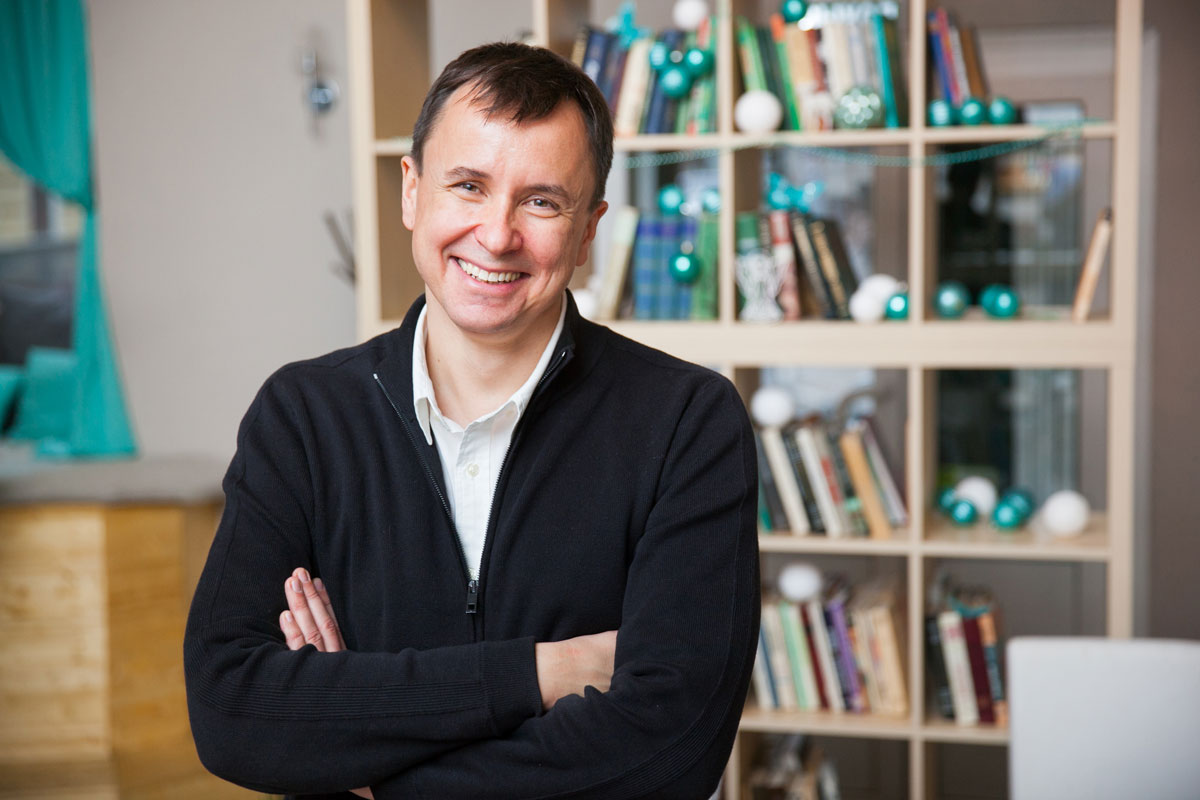Andrei Okounkov

Professor of Mathematics
Princeton University

A series of three lectures entitled Applied Noncommutative Geometry

Abstract: Noncommutative projective geometry deals with noncommutative algebras that, in a certain technical sense, are just as good as polynomials.

Their study was begun by M. Artin and W. Schelter over twenty years ago and grew into a rich and fascinating subject since. In these lectures, I will explain some applications of this theory to such basic mathematical objects as linear difference equations. These difference equations are of direct probabilistic interest (which will be also explained in the lectures) and thus noncommutative algebraic surfaces shed light on the behavior of random stepped surfaces.

Lecture I: https://youtu.be/YF2dYDToe38

Lecture II: https://youtu.be/fZqitUVVFwM

Lecture III: https://youtu.be/fzJEjYxsd2o

Top of page

## 2007-2008 Milliman Lectures

###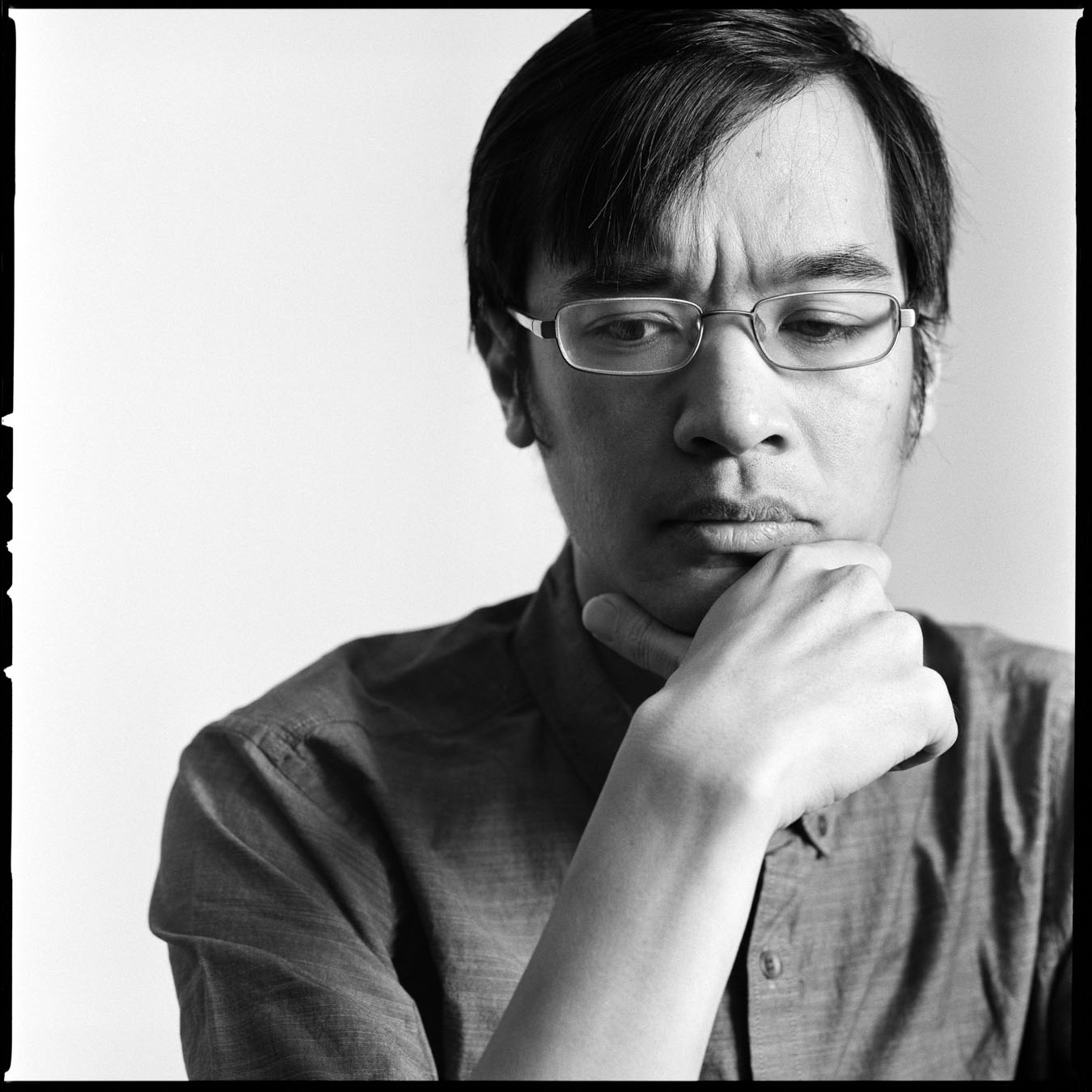Terence Tao

Professor of Mathematics
University of California, Los Angeles

A series of three lectures entitled Recent Developments in Arithmetic Combinatorics

Lecture I: Additive Combinatorics and the Primes
Given a set A of N integers, how many additive patterns (e.g. arithmetic progressions of length three) does A have? The answer depends of course on the nature of A. At one extreme are sets with very strong additive structure, such as the arithmetic progression {1,..., N}, which tend to have many additive patterns. At the other extreme are "random" sets A, which tend to have relatively few additive patterns. Understanding and classifying these two extremes, and the extent to which an arbitrary set lies between these extremes, is a major topic of additive combinatorics today. Recent progress on these questions has led to applications in number theory, particularly regarding the question of detecting additive patterns in the primes; in particular, I will discuss my result with Ben Green that the primes contain arbitrarily long arithmetic progressions.

Video: https://youtu.be/Lz3JG_V6f-Q

Lecture II: Additive Combinatorics and Random Matrices
The theory of random continuous matrices (such as the gaussian unitary ensemble or GUE), and in particular the study of their eigenvalues, is an intensively studied and well-understood subject. In contrast, the theory of random discrete matrices, such as the Bernoulli ensemble in which each entry of the matrix equals +1 and -1 with equal probability, is only just now being developed. Much of the recent progress relies on an understanding of the distribution of discrete random walks, and in particular on solving the inverse Littlewood-Offord problem. This in turn requires the use of tools from additive combinatorics, such as the geometry of multidimensional arithmetic progressions, and Freiman's inverse theorem. I will survey these developments, which include joint work of myself with Van Vu.

Lecture III: Sum-product Estimates, Expanders, and Exponential Sums
Consider a finite set A of elements in a ring or field. This set might be "almost closed under addition", which for instance occurs when A is an arithmetic progression such as {1,..., N}. Or it may be "almost closed under multiplication", which for instance occurs when A is a geometric progression such as {1, a,..., aN}. But it is difficult for A to be almost closed under addition and multiplication simultaneously, unless it is very close to a subring or subfield. The emerging field of sum-product estimates in arithmetic combinatorics seeks to establish strong inequalities to quantify this phenomenon. Recent breakthroughs in this area by Bourgain and coauthors, based primarily on Fourier analysis and additive combinatorial tools, have yielded new exponential sum and sieve estimates in number theory, new randomness extractors in computer science, and new constructions of expander graphs in combinatorics. This lecture will be a survey of several of these developments.

Video: https://youtu.be/iLvAG2CeBN8

Top of page

## 2006-2007 Milliman Lectures

###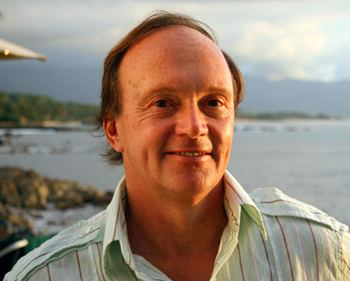Richard Hamilton

Professor of Mathematics
Columbia University

Lecture I: Geometrization of Three-Dimensional Spaces
Video: https://youtu.be/BuSLy5OQctk

Lecture II: The Ricci Flow on Kahler Manifolds
Video: https://youtu.be/EMxSoOL-DIs

Lecture III: Estimates for the Ricci Flow
Video: https://youtu.be/kuFdcvi5v54

Top of page

## 2005-2006 Milliman Lectures

###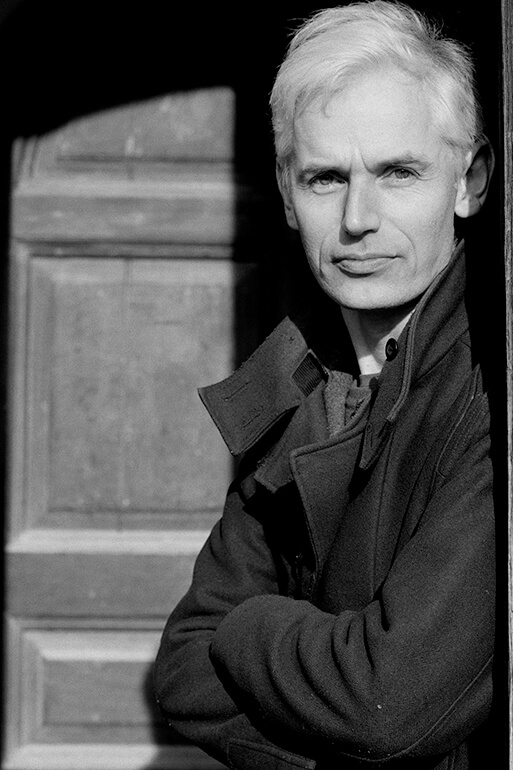Timothy Gowers

Rouse Ball Professor of Mathematics
Cambridge University

Lecture I: Some Theorems and Open Problems in Arithmetic Combinatorics
Arithmetic combinatorics is the name that has been given to a thriving new area of mathematics, or, to be more accurate, a new and very interesting fusion of old areas. I shall give an overview of the area and try to explain what combinatorics, harmonic analysis, and number theory have to offer each other.

Lecture II: Discrete Fourier Analysis: Its Uses and Limitations
This lecture will be about one of the main techniques in arithmetic combinatorics: the use of the discrete Fourier transform. I shall outline how it can be used to prove two central theorems in the subject, namely Roth's theorem on arithmetic progressions, and Freiman's theorem on the structure of sets with small sumsets. Both these theorems result in major open problems, and I shall try to explain their difficulty by highlighting what it is that we do not yet understand about Fourier analysis.

Lecture III: Discrete Fourier Analysis: Its Uses and Limitations
In this lecture I shall try to give some idea about the proofs of two celebrated results about arithmetic progressions: Szemerécdi's theorem, which states that every dense set of integers contains arbitrarily long arithmetic progressions, and the Green-Tao theorem, which tells us that the same is true of the set of primes. Both these results involve situations where conventional Fourier analysis is inadequate, but recently discovered "polynomial" generalizations can be used instead. Much remains to be understood: if time permits, I shall speculate about what arithmetic combinatorics may look like in twenty years' time.

Top of page

## 2004-2005 Milliman Lectures

###Luis Caffarelli

Sid Richardson Chair holder, Professor in Mathematics
University of Texas at Austin, and the Texas Institute for Computations and Applied Mathematics

A series of three lectures entitled Free Boundary Problems of Obstacle Type

Abstract: Free boundary problems of obstacle type became of interest during the development of the theory of Variational Inequalities in the late 1960s, and applications surfaced in many areas of applied math, probability and geometry.

From the variational point of view, the problem consists of minimizing some variational integral , among those configurations staying above a given graph. From the probabilistic point of view, it concerns an optimal stopping time problem that gives rise to a Hamilton-Jacobi-Bellman equation.

Typical examples of the variational integrals involve those giving rise to second order linear or non linear equations (Laplacian, minimal surfaces, p-Laplacian), fourth order problems like the bi-Laplacian (clamped plates), or the fractional Laplacian for instance for boundary control problems or optimal stopping times for Levi processes. We will discuss matters of regularity, stability, and the geometric properties of the contact set and its free boundary.

Lecture I: We will discuss what is a free boundary problem, in particular one of obstacle type, describe different areas, from geometry to fluid dynamics to probability in which they appear, and what type of information we seek.

Lecture II: We will give a more detailed discussion of regularity and geometric behavior of solutions to these types of problems, as well as its interpretations, in particular in connection with recent work on non-local variational inequalities.

Lecture III: We will discuss in greater detail the main techniques to tackle this type of problem, in particular "convexity" properties of solutions, and the role of monotonicity formulas.

Top of page

## 2003-2004 Milliman Lectures

###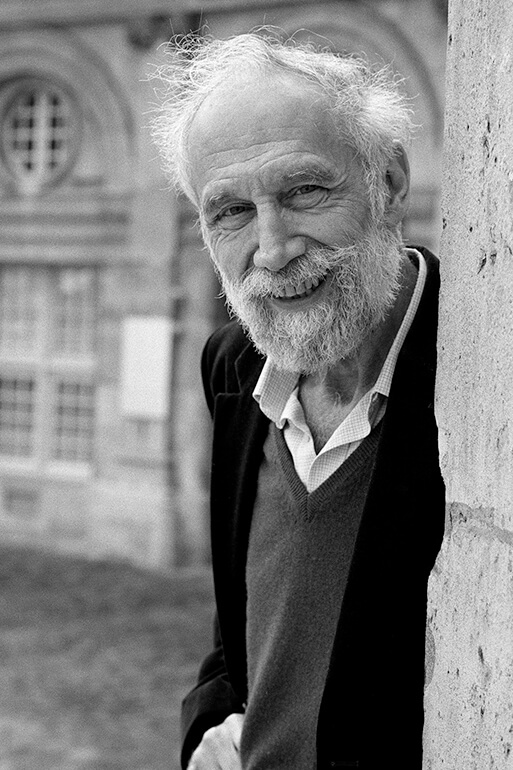Alain Connes

Professor of Mathematics
Collège de France, and Institut des Hautes Études Scientifiques

A series of three lectures entitled Non-Commutative Geometry

Abstract: Non-commutative geometry has its roots in the following theorem of Gelfand: if X is a (locally) compact Hausdorff space and C(X) is the C*-algebra of continuous complex-valued functions on X, then X may be recovered as the space of maximal ideals of C(X). More precisely, the category of commutative C*-algebras is equivalent to the category of locally compact Hausdorff spaces.

Gelfand's Theorem allows one to transport the usual geometric notions to corresponding algebraic properties and features of the ring C(X). For example, complex vector bundles on X correspond to finitely generated projective modules over C(X), and the topological K-theory of X is isomorphic to the algebraic K-theory of C(X).

The basic idea behind non-commutative geometry is allow C*-algebras that are not necessarily commutative to play the role of "functions" on spaces that are not necessarily Hausdorff.

Among the naturally occurring spaces with obvious geometric meaning that fail to be Hausdorff are:

• the space of leaves of a foliation
• the space of irreducible representations of a discrete group
• the space of Penrose tilings of the plane
• the Brouillon zone in the quantum Hall effect
• phase space in quantum mechanics
• space-time
• the space of Q-lattices in Rn

Far-reaching non-commutative extensions of classical geometric concepts such as measure theory, topology, differential geometry, and Riemannian geometry have been obtained with varying degrees of perfection. These extensions allow the usual geometric notions to be used to analyze spaces such as those above.

These three talks will provide an introduction to, and overview of, non-commutative geometry. Connections with other branches of mathematics and physics will be emphasized.

Top of page

## 2002-2003 Milliman Lectures

###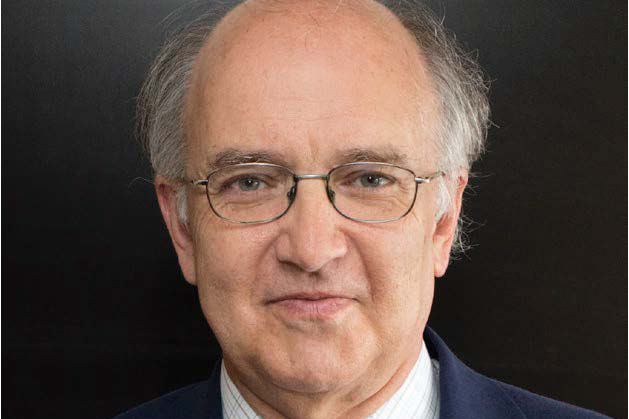János Kollár

Professor of Mathematics
Princeton University

Lecture I: What is the Biggest Multiplicity of a Root of a Degree d Polynomial?

Lecture II: What are the Simplest Algebraic Varieties?

Lecture III: Rational Connected Varieties over Finite Fields

Top of page

## 2001-2002 Milliman Lectures

###Peter Sarnak

Professor of Mathematics
Institute for Advanced Study, Princeton, and New York University

A series of three lectures entitled Familes of L-functions and Applications

Abstract: L-functions, starting from Riemann's zeta function and continuing to the modern automorphic L-function have played a central role in number theory. These lectures will focus on recent developments in the analytic theory of such functions. A key technique which is at the heart of these advances is based on the formation and analysis of families of L-functions. There are numerous applications of these developments to number theory, combinatorics and mathematical physics. We will describe two in detail. One is the solution of Hilbert's eleventh problem which asks about the representability of integers in a number field by an integral quadratic form. The other is to eigenfunctions on an arithmetic surface and in particular problems in quantum chaos.

Top of page

## 2000-2001 Milliman Lectures

###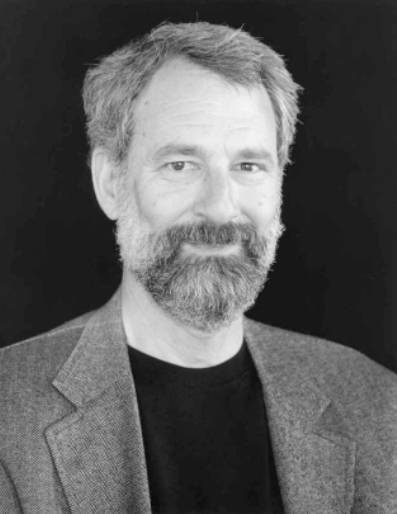Charles Fefferman

Professor of Mathematics
Princeton University

A series of three lectures entitled Unsolved Problems of Fluid Dynamics

Abstract: The talks will state some of the main unsolved problems on the Euler and Navier-Stokes equations for incompressible fluids, and sketch the proofs of some of the main results known so far on these and related equations. I hope to get through the main ideas in the proofs of the Beale-Kato-Majda theorem and results of Constantin, Majda and me on breakdown of Euler solutions, the work of Sheffer, Caffarelli-Kohn-Nirenberg and F. H. Lin on Navier-Stokes, and recent results by D. Cordoba and me on breakdown scenarios. Also, I hope to state precisely some problems arising from Kolmogorov's ideas on turbulence.

Top of page

## 1999-2000 Milliman Lectures

###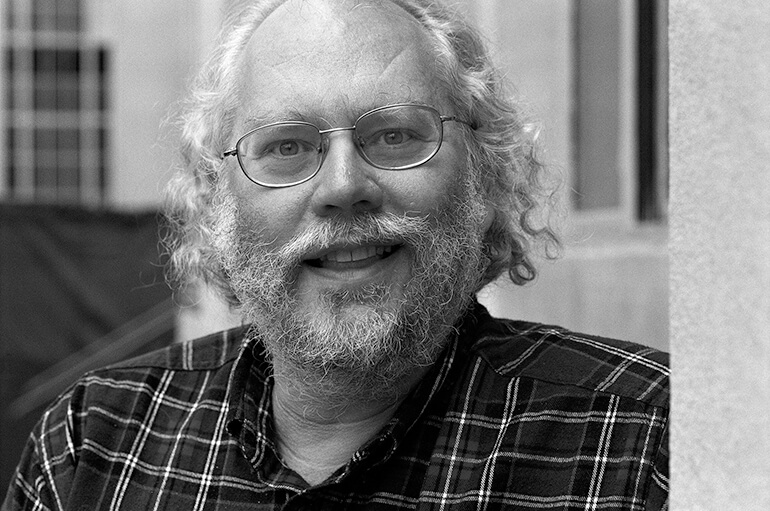Peter Shor

AT&T Research

Lecture I: Quantum Algorithms
Quantum computers are hypothetical devices that use the principles of quantum mechanics to perform computations. For some difficult computational problems, including the cryptographically important problems of prime factorization and finding discrete logarithms, the best algorithms known for classical computers are exponentially slower than ones known for quantum computers. Although they have not yet been built, quantum computers do not appear to violate any fundamental principles of physics. I will explain how quantum mechanics provides this extra computational power, and show how Fourier transforms lets quantum computers find periodicity in situations classical computers cannot. Finally, I show how large integers can be factored by finding the periodicity of an exponentially long sequence.

Lecture II: Quantum Error Correction
One of the main difficulties in building quantum computers is in manipulating coherent quantum states without introducing errors or losing coherence. This problem can be alleviated by the use of quantum error correcting codes. Until these codes were discovered, it was widely believed that quantum computers could not be made resistant to errors - the argument was that to detect an error, a quantum computer's state would have to be measured, which by the laws of quantum mechanics would perturb the computer's state and thus disrupt the computation. Quantum error correcting codes get around this by encoding the data so that errors can be measured without disturbing the computational state. I will explain how these codes work, and briefly sketch how they can be used to make quantum computes extremely reliable via software if they can be built with only moderately reliable hardware.

Lecture III: Quantum Information Theory
One of the most remarkable papers of this century was the 1948 paper in which Shannon laid out the fundamentals of information theory, giving formulae for the compressibility of data and for the information-carrying capacity of a channel. Only in the last ten years, however, have researchers started to investigate what happens to the theorems of information theory in a quantum mechanical setting. We will define quantum channels, and present what is known about the analogs of Shannon's theorems in the quantum setting. Aside from knowledge of basic quantum mechanics (the necessary part of which I plan to cover in the first lecture), these lectures should be independent.

Top of page

## 1998-1999 Milliman Lectures

###Clifford Taubes

Professor of Mathematics
Harvard University

A series of three lectures titled New Geometry in Four Dimensions

Abstract: The more we learn about the classification of smooth (even simply connected) four dimensional manifolds, the less we understand; to the point where today, we have no viable conjectures. My lectures will focus first on how we got to this amusing state (the Seiberg-Witten invariants helped) - here you will see lots of 4-dimensional manifolds which sit just across the boundary of what we understand. Then, I will discuss a particular approach for moving across the frontier, this being a curious four dimensional generalization of Morse theory in dimension 3 which mixes Riemannian, symplectic and holomorphic geometry.

Top of page

## 1997-1998 Milliman Lectures

###Robert MacPherson

Professor of Mathematics

A series of three lectures entitled:

Lecture I: Counting Faces of Polyhedra

Lecture II: Fixed Points in Spaces of Lattices

Lecture III: Topology of Spaces with Torus Actions

Top of page

## 1996-1997 Milliman Lectures

###Ingrid Daubechies

Professor of Mathematics
Princeton University

Lecture I: Wavelets: An Overview, with Recent Applications
Wavelets have emerged in the last decade as a synthesis from many disciplines, ranging from pure mathematics (where forerunners were used to study singular integral operators) to electrical engineering (quadrature mirror filters), borrowing in passing from quantum physics, from geophysics and from computer aided design. The first part of the talk will present an overview of the ideas in wavelet theory, in particular wavelet bases. The second part of the talk will discuss some recent applications, ranging from loss-less as well as lossy image compression or speech analysis to nonlinear approximation methods.

Lecture II: Subdivision Schemes
Subdivision schemes are used in computer aided design for the construction of locally adaptable curves and surfaces. A "good" scheme can lead to surfaces or curves that look quite smooth. This talk will discuss mathematical techniques to study the regularity of these curves and surfaces. In the "equally spaced" case, this is all well understood. The corresponding techniques in the non-equally spaced case are under development now; the talk will end with a presentation of some work in progress on this topic.

Lecture III: Using Transfer Operators to Find the Regularity of Refinable Functions
A function f(x) is refinable if it can be written as a linear combination of the dilated and translated copies f(2x -n) of f. Such functions come up naturally in the construction of wavelet bases and in equally spaced subdivision schemes. Many techniques exist to find the regularity of such functions; for most, the complexity of the computation depends crucially on the number of coefficients is the refinement equation. This talk will present a link with transfer operators for the doubling operator, used in an estimation method for the regularity that can handle even infinite numbers of coefficients.

Top of page

## 1995-1996 Milliman Lectures

###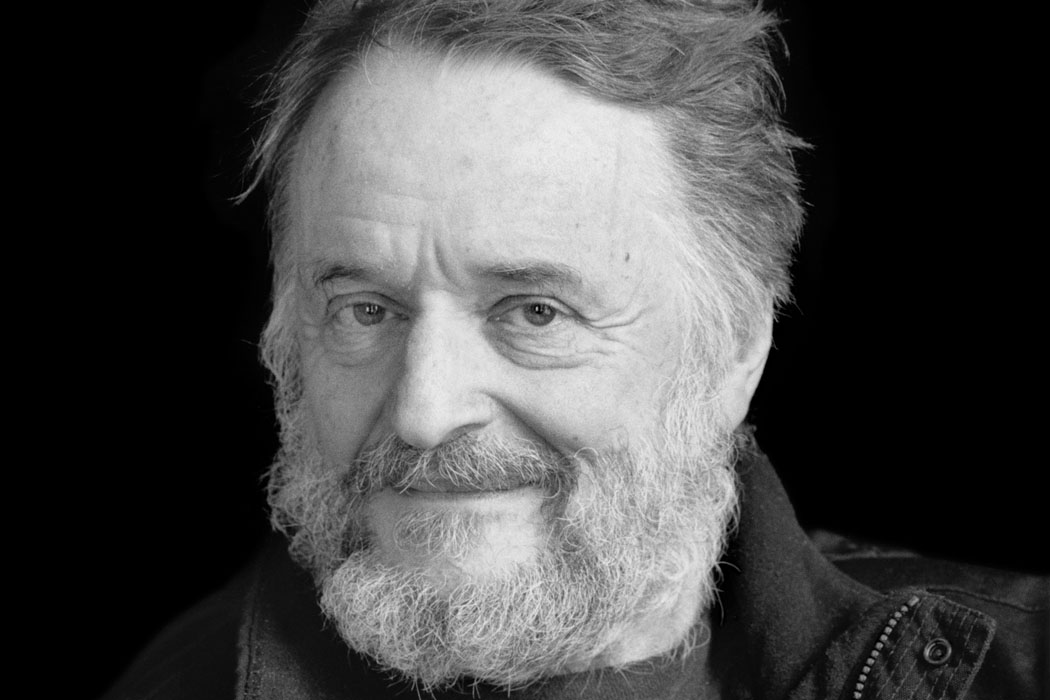John Conway

Professor of Mathematics
Princeton University

A series of three lectures entitled On Games, Codes, and Balls

Lecture I: Games and Numbers
How adding up games helps to understand numbers.

Lecture II: Codes and Numbers
The mysterious arithmetic of lexicographic codes.

Lecture III: Spheres and Clusters
How to pack a big box with small balls.

Top of page

## 1994-1995 Milliman Lectures

###Gil Kalai

Professor of Mathematics
Hebrew University of Jerusalem

Lecture I: Combinatorics and Convexity

Lecture II: Simple Polytopes

Lecture III: The Combinatorics of the Simplex Algorithm

Top of page

## 1993-1994 Milliman Lectures

###Louis Nirenberg

Professor of Mathematics
New York University

A series of three lectures entitled Variational Methods, the Maximum Principle and Related Topics

Top of page

## 1992-1993 Milliman Lectures

###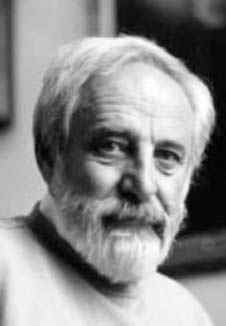Raoul Bott

Professor of Mathematics
Harvard University

A series of three lectures entitled On some Physics-Inspired Aspects of Geometry and Topology

Abstract: General relativity and the Quantum Theory have been two of the great theoretical driving forces of this century - not only of Physics but also of Mathematics. Relativity is by its very nature geometric and, dealing as it does with the very distant, it has natural links to topology. The Quantum theory on the other hand is traditionally more associated with questions in functional analysis. Still this theory has profound and rather surprising relations with topology and in these lectures I hope to explore some of these.

Top of page

## 1991-1992 Milliman Lectures

###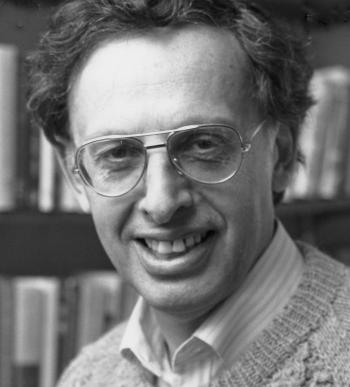Elias M. Stein

Professor of Mathematics
Princeton University

A series of three lectures entitled Oscillatory Integrals in Analysis

Top of page

## 1990-1991 Milliman Lectures

###Barry Mazur

Professor of Mathematics
Harvard University

A series of three lectures entitled In Search of the Monster

Top of page

## 1989-1990 Milliman Lectures

###Richard Stanley

Professor of Mathematics
Massachusetts Institute of Technology

A series of three lectures entitled Some Applications of Algebra to Combinatorics

Abstract: We will survey a number of applications of algebra to some problems in combinatorics dealing with extremal set theory, partially ordered sets, enumeration, and convex polytopes. No special knowledge of algebra or combinatorics will be necessary to understand these talks. We begin with teh simplest linear algebra and build up more and more sophisticated machinery as it is required. Some of the topics covered are the following:
• the use of linear algebra to prove the classical Sperner theorem (which determines the largest collection A of subsets of an n-element set such that no member of A is a subset of another) and related results,
• the use of finite group theory to prove some variations of the Sperner theorem, including the unimodality of the q-binomial coefficients,
• a proof of a number-theoretic conjecture of Erdos and Moser using the representation theory of the Lie algebra sl(2,C),
• a proof of the Upper Bound Conjecture for Spheres using the theory of Cohen-Macaulay rings, and
• a proof of McMullen's g-conjecture for convex polytopes based on the theory of toric variaties and the hard Lefschetz theorem.

Top of page

## 1988-1989 Milliman Lectures

###Michael Artin

Professor of Mathematics
Massachusetts Institute of Technology

A series of three lectures entitled Deformation of Polynomial Rings

Top of page

## 1987-1988 Milliman Lectures

###Peter Lax

Professor of Mathematics
New York University

A series of three lectures entitled Wave Propagation

Top of page

## 1986-1987 Milliman Lectures

###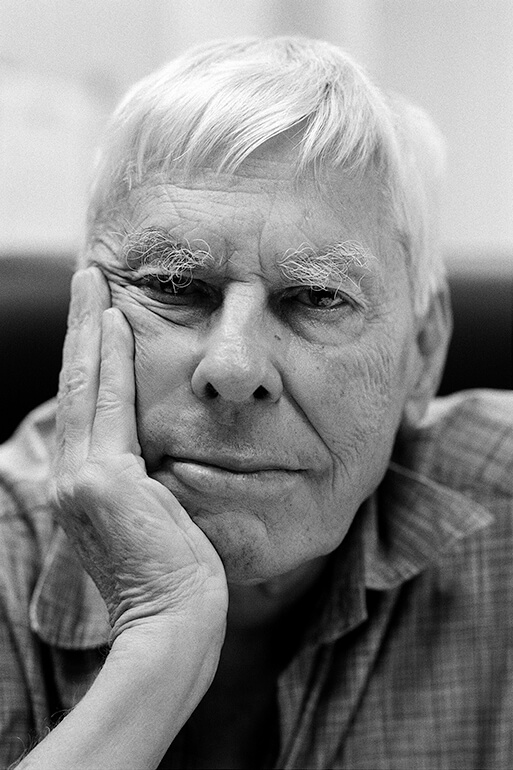Stephen Smale

Professor of Mathematics
University of California, Berkeley

A series of three lectures entitled Structure and Complexity Theory of Algorithms for Nonlinear Equations

Top of page

## 1986 Milliman Lectures

###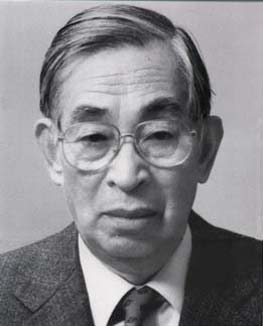Kiyoshi Itô

Professor of Mathematics
Kyoto University

A series of four lectures entitled Diffusions in Infinite Dimensional Spaces

The lectures were in conjunction with the Workshop in Markov Processes which took place at the University of Washington from June 23 to July 2, 1986.

Top of page

## 1985 Milliman Lectures

###Hillel Furstenberg

Professor of Mathematics
Hebrew University

A series of three lectures entitled Ergodic Theory and Combinatorics

Top of page

## 1984-1985 Milliman Lectures

###Frank Adams

Lowndean Professor of Astronomy and Geometry
University of Cambridge

Top of page

## 1983-1984 Milliman Lectures

###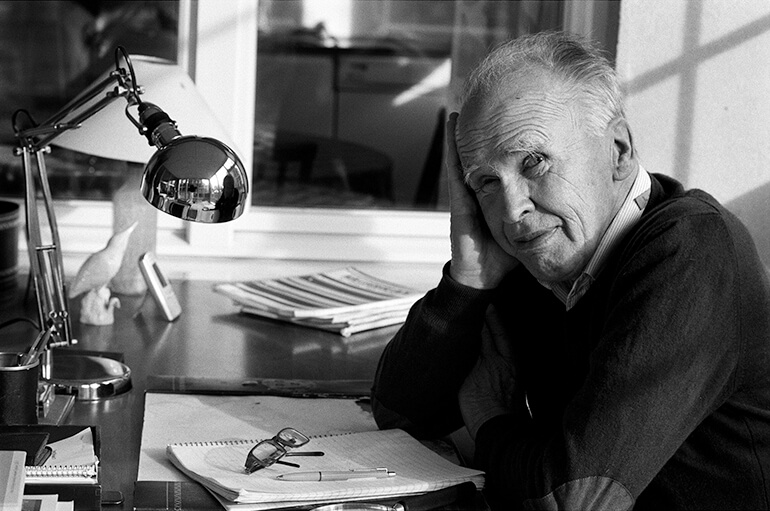Lennart Carleson

Professor of Mathematics
Uppsala University

A series of two lectures entitled On information theory and the support of harmonic measure and On iterations of quadratic polynomials.

Top of page